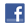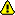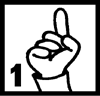New account
4 million accounts created!

• Home
• Report a bug

•

Learn Dutch > Dutch lessons and exercises > Dutch test #22029> Other Dutch exercises on the same topic: Numbers [Change theme] > Similar tests: - Numbers 1 to 10 - Numbers 1 to 99 - Maths & numbers - Janssens (38)- Coffee - Numbers 1 to 20 - Numbers - Numbers-Phone numbers - Maths > Double-click on words you don't understand

Write down the result.Twitter Share
Dutch exercise "Additions" created by mariebru with The test builder. [More lessons & exercises from mariebru]Please log in to save your progress.

To insert special letters:

1.+=

2.=

3.+=

4.++=

5.+=

6.+++7.+=

8.=

9.++=

10.+=

11.++=

12.+=

13.+++=

14.++=

15.++=

16.+++=

17.+++=

18.++=

19.+++=

20.++=

End of the free exercise to learn Dutch: Additions
A free Dutch exercise to learn Dutch.
Other Dutch exercises on the same topic : Numbers | All our lessons and exercises